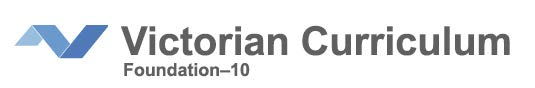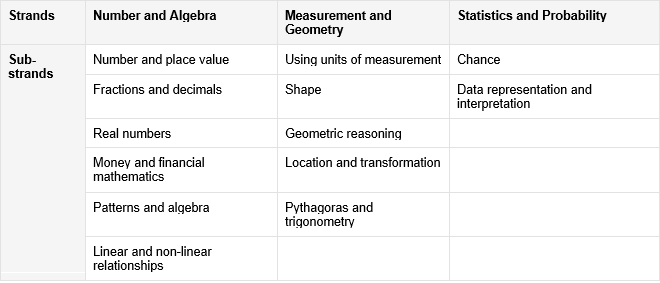# Mathematics at featherbrook

The Featherbrook College Mathematics provision is drawn from the mandated Victorian Curriculum which is organised by the three strands of Number and Algebra, Measurement and Geometry, and Statistics and Probability. Each strand is organised by sub-strands. Sub-strands group content descriptions under an appropriate concept, to provide both a focus and a clear sequence for the development of related concepts and skills within strands and across levels.Teachers place an emphasis on developing student knowledge, application of key skills and concepts, and positive dispositions towards mathematics through delivery of high quality challenging mathematical tasks that incorporate the four mathematical proficiencies. The proficiencies of Understanding, Fluency, Problem Solving and Reasoning are fundamental to learning mathematics and working mathematically. The proficiencies are applied across all three strands Number and Algebra, Measurement and Geometry, and Statistics and Probability.

Students in the primary years have daily mathematics sessions, whilst secondary students attend a minimum of four mathematics sessions per week. Specialist and electives subjects make connections and develop relationships between mathematics and other disciplines.

### Resource Provision

Each learning space is equipped with purposefully selected physical manipulatives to support the classroom program and age and developmental stage of the students. Teachers draw upon the following quality assured resources and assessment tools to plan and deliver highly engaging and impactful lessons

DET Numeracy Portal including the Mathematics Teaching Toolkit, Mathematics Curriculum Companion and Birth to Level 10 Numeracy Guide

VCAA Formative Assessment Guide and Numeracy Learning Progressions

### Number and Algebra

Number and Algebra are developed together and each enriches the study of the other. Students apply number sense and strategies for counting and representing numbers. They explore the magnitude and properties of numbers. Students apply a range of strategies for computation and understand the connections between operations. They recognise patterns and understand the concepts of variable and function. They build on their understanding of the number system to describe relationships and formulate generalisations. Students recognise equivalence and solve equations and inequalities. They apply their number and algebra skills to conduct investigations, solve problems and communicate their reasoning.

### Measurement and Geometry

Measurement and Geometry are presented together to emphasise their relationship to each other, enhancing their practical relevance. Students develop an increasingly sophisticated understanding of size, shape, relative position and movement of two-dimensional figures in the plane and three-dimensional objects in space. They investigate properties and apply their understanding of them to define, compare and construct figures and objects. They learn to develop geometric arguments. Students make meaningful measurements of quantities, choosing appropriate metric units of measurement. They build an understanding of the connections between units and calculate derived measures such as area, speed and density.

### Statistics and Probability

Statistics and Probability develops initially in parallel, with the curriculum progressively building links between them. Students recognise and analyse data and draw inferences. They represent, summarise and interpret data and undertake purposeful investigations involving the collection and interpretation of data. Students recognise variation, assess likelihood and assign probabilities using experimental and theoretical approaches. They develop an increasingly sophisticated ability to critically evaluate chance and data concepts and make reasoned judgments and decisions, as well as building skills to critically evaluate statistical information and develop intuitions about data.

### Learning in Mathematics

The proficiencies of Understanding, Fluency, Problem Solving and Reasoning are fundamental to learning mathematics and working mathematically. They are applied across all three strands Number and Algebra, Measurement and Geometry, and Statistics and Probability.

Understanding refers to students building a robust knowledge of adaptable and transferable mathematical concepts and structures. Students make connections between related concepts and progressively apply the familiar to develop new ideas. They develop an understanding of the relationship between the ‘why’ and the ‘how’ of mathematics. Students build understanding when they:

• connect related ideas

• represent concepts in different ways

• identify commonalities and differences between aspects of content

• describe their thinking mathematically

• interpret mathematical information.

Fluency describes students developing skills in choosing appropriate procedures, carrying out procedures flexibly, accurately, efficiently and appropriately, and recalling factual knowledge and concepts readily. Students are fluent when they:

• make reasonable estimates

• recognise robust ways of answering questions

• choose appropriate methods and approximations

• recall definitions and regularly use facts,

• can manipulate expressions and equations to find solutions

Problem-solving is the ability of students to make choices, interpret, formulate, model and investigate problem situations, select and use technological functions and communicate solutions effectively. Students pose and solve problems when they:

• use mathematics to represent unfamiliar or meaningful situations

• apply their existing strategies to seek solutions

• verify that their answers are reasonable.

Reasoning refers to students developing an increasingly sophisticated capacity for logical, statistical and probabilistic thinking and actions, such as conjecturing, hypothesising, analysing, proving, evaluating, explaining, inferring, justifying, refuting, abstracting and generalising. Students are reasoning mathematically when they:

• explain their thinking

• deduce and justify strategies used and conclusions reached

• adapt the known to the unknown

• transfer learning from one context to another

• prove that something is true or false

• make inferences about data or the likelihood of events

• compare and contrast related ideas and explain their choices.